Importance: Medium ✭✭
 Author(s): Erdos, Paul Nesetril, Jaroslav
 Subject: Graph Theory » Coloring » » Edge coloring
 Keywords:
 Recomm. for undergrads: no
 Posted by: fhavet on: March 1st, 2013

A strong edge-colouring of a graphis a edge-colouring in which every colour class is an induced matching; that is, any two vertices belonging to distinct edges with the same colour are not adjacent. The strong chromatic index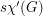is the minimum number of colours in a strong edge-colouring of.

ConjectureThe conjectured bounds would be sharp. Whenis even, expanding each vertex of a-cycle into a stable set of sizeyields such a graph with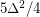edges in which the largest induced matching has size. A similar construction achieves the bound whenis odd.

Greedy colouring the edges yields. Using probabilistic methods, Molloy and Reed~[MoRe97] proved that there is a positive constantsuch that, for sufficiently large, every graph with maximum degreehas strong chromatic index at most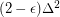.

The greedy bound proves the conjecture for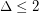. For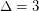, the conjectured bound of 10 was proved independently by Hor\'ak, He, and Trotter[HHT] and by Andersen [A]. For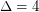, the conjectured bound is 20, and Cranston [C] proved that 22 colours suffice.

For a bipartite graph, Faudree et al. [FGST] conjectured that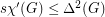. This is implied by the stronger conjecture due to Kaiser.

Conjecture   Letbe a bipartite graph such that every vertex in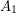has degree at mostand every vertex inhas degree at most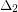. Then.

## Bibliography

[A] L. D. Andersen. The strong chromatic index of a cubic graph is at most 10. Discrete Math., 108(1-3):231--252, 1992.

[C] D. W. Cranston. Strong edge-coloring of graphs with maximum degree 4 using 22 colors. Discrete Math., 306(21):2772--2778, 2006.

[FGST] R. J. Faudree, A. Gyárfás, R. H. Schelp, and Zs. Tuza. Induced matchings in bipartite graphs. Discrete Math., 78(1-2):83--87, 1989.

[HHT] P. Horák, Q. He, and W. T. Trotter. Induced matchings in cubic graphs. J. Graph Theory, 17(2):151--160, 1993.

* indicates original appearance(s) of problem.